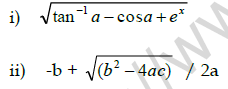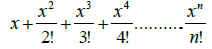# CBSE Class 11 Computer Science Question Paper Set M Solved

Read and download CBSE Class 11 Computer Science Question Paper Set M Solved designed as per the latest question paper pattern and Class 11 examination guidelines issued by CBSE, NCERT and KVS. The past year Question Papers for Class 11 Computer Science have been provided with solutions which will help students to assess their performance and find out topics in Computer Science grade 11 which they need to improve to get better marks in Standard 11 exams. After solving these last year papers also refer to solved Sample Papers for Class 11 Computer Science available on our website to build strong understanding of the subject

## Computer Science Question Paper Class 11

Students can refer to the below Class 11 Computer Science Question Paper designed to help students understand the pattern of questions that will be asked in Grade 11 exams. Please download CBSE Class 11 Computer Science Question Paper Set M Solved

### Computer Science Class 11 Question Paper

1. a. What is a Cache memory? Why it is considered crucial for a microprocessors performance?

b. What do you mean by Bluetooth? Explain.

c. What is the difference between CISC and RISC Computers?

d. Explain Parallel Port.

e. What is the difference between RAM and ROM?

f. What are the basic characteristics of a Microprocessor?

g. What do you mean by a port in a computer? Explain InfraRed Port.

h. Write a note on:

i) Blu Ray Disc    ii) Hard Disks

2. a. What does the file iostream.h consist of?

b. What do you mean by cascading of I/O operators? Give an example.

c. What is the difference between a keyword and an identifier?

3. a. Consider the following two C++ statements. Are they equivalent? Explain.
char x = 'A' ;
char x = 65 ;

b. In how many ways can a variable be declared in C++? Give examples.

c. Define the following terms:

i) Data type modifier ii) Reference variable

4. a. What do you mean by implicit and explicit type conversion? Explain.

b. Use conditional operator, to represent the following in C++ If amount>30000, tax=5000 otherwise tax=2500.

c. Given the following expressions:

(a) val = 80 (b) val == 80
i) How are these two different?
ii) What will be the result of (a) and (b) if the value of val is 90 initially?

d. Construct logical expressions to represent the following conditions:

i) salary is greater than or equal to 30000 but less than 35000
ii) x is even and more than 50.

e. Write the output of the following:

i) int a, b, c=12;
a=(b=7, b+2);
cout<< ”A=” <<a<< ‟\n‟<< “B=” <<b;

ii) int a=5;
int b =++a + 50;
int c = a-- + 40;
cout<<”b=” <<b << “\n” << “c=” <<c;

f. Write the corresponding C++ expressions for the following mathematical expressions:5. a. What is meant by exit-controlled loop? Which C++ loops are exit-controlled?

b. Briefly explain goto statement in C++ with suitable example.

c. What is the significance of a break statement in a switch statement? Explain with an example.

d. Rewrite the following code using while loop:

int x, sum;
for(x=5, sum=0; x<25; x+=5)
sum+=x;
cout<<”sum= “<<sum;

e. Predict the output of the following C++ program:

#include<iostream.h>
void main()
{
for(int i=0; i<2; i++)
{ for(int j=1; j<=12; j=j+3)
cout<< j << ” “;
cout<<endl;
} }

f. Rewrite the following program after removing the syntactical error(s), if any. Underline each correction.

#include<iostream.h>
void main( )
{ int n1=5;
do
{ n2 =* n1;
cout<n2;
} while(n1<10) }

g. Rewrite the following code fragment using switch…case statement :

char gr;
if (gr==„A‟)
else if (gr==„B‟)
else if (gr==„C‟)
else
cout<<”Fail”;

6. a. Write a program to input three integers and print the smallest of the three.

b. Write a program to print the following series.
1 -4 7 -10 ………… .-40

c. Write a C++ program to generate the following pattern:
5 4 3 2 1
5 4 3 2
5 4 3
5 4
5

d. Write a program to print sum of even numbers and sum of odd numbers from a list of numbers entered by the user. The list terminates when the number entered is zero.

7. a. Write a program to accept monthly salary from the user. Find and display Bonus with the help of following rules:

Monthly Salary                                          Bonus

50001 onwards                             30% of Monthly Salary
30001 - 50000                              20% of Monthly Salary
10001 – 30000                             10% of Monthly Salary
0 – 10000                                      5% of Monthly Salary

b. Write a menu driven Program in C++ which displays the following menu:

1. Check positive or negative
2. Display the factors
3. Exit

The Program reads a choice (1, 2 or 3) from the user and performs the following : If the user enters a choice 1, read a non zero integer from the user and check if it is positive or negative.
If the choice is 2, read an integer from the user and display all its factors.
If the choice is 3, the Program terminates.
Any other choice should display an error message.
The menu should keep on displaying till the user wants it .

c. Write a program to accept the value of x and n and display the sum of the following series:d. Write a program to accept an integer and check whether it is an Armstrong number or not and display proper message as output.

e.g . 153 is an Armstrong number, ( 153 = 13 + 53+ 33)

## Tags:

Click for more Computer Science Study Material
 CBSE Class 11 Computer Science Question Paper Set H CBSE Class 11 Computer Science Question Paper Set R Solved CBSE Class 11 Computer Science Question Paper Set L Solved CBSE Class 11 Computer Science Question Paper Set G CBSE Class 11 Computer Science Question Paper Set S Solved CBSE Class 11 Computer Science Question Paper Set M Solved CBSE Class 11 Computer Science Question Paper Set E CBSE Class 11 Computer Science Question Paper Set T Solved CBSE Class 11 Computer Science Question Paper Set K CBSE Class 11 Computer Science Question Paper Set N Solved CBSE Class 11 Computer Science Question Paper Set B CBSE Class 11 Computer Science Question Paper Set U Solved CBSE Class 11 Computer Science Question Paper Set J CBSE Class 11 Computer Science Question Paper Set O Solved CBSE Class 11 Computer Science Question Paper Set D CBSE Class 11 Computer Science Question Paper Set V Solved CBSE Class 11 Computer Science Question Paper Set I CBSE Class 11 Computer Science Question Paper Set P Solved CBSE Class 11 Computer Science Question Paper Set C CBSE Class 11 Computer Science Question Paper Set F CBSE Class 11 Computer Science Question Paper Set Q Solved CBSE Class 11 Computer Science Question Paper Set A

## Latest NCERT & CBSE News

Read the latest news and announcements from NCERT and CBSE below. Important updates relating to your studies which will help you to keep yourself updated with latest happenings in school level education. Keep yourself updated with all latest news and also read articles from teachers which will help you to improve your studies, increase motivation level and promote faster learning

### How To Solve Unseen Passages In English

Unseen passages may contain one or many paragraphs. This is one of the important yet easy parts for a student to get marks. Students should thoroughly study and understand the passage to answer the related questions. The unseen passages are there just to test the...

### Score well in Class 12 English Boards Exam

12th Board exams are an important part of students' lives. The marks obtained in the board exam decide the college in which one can study. In class 12 the syllabus of each and every subject increases vastly and it is difficult to cover up every point. In English also...

### CBSE Class 10 Revised Syllabus

Last year CBSE had to reduce the syllabus because of the pandemic situation but it was not very effective because there were no examinations. This year to avoid any confusion and conflict, CBSE has decided to reduce the syllabus into term 1 and term 2. 50 percent of...

### MCQ Question based CBSE examination

For 2021-22 CBSE has launched MCQ question-based examination for Term 1 & Term 2 board examinations. The entire syllabus has been divided into two parts each including 50% of the entire syllabus. To score well, students must practice as per the new CBSE term-wise...

### CBSE Board Examination Scheme of Assessment 2021 2022

A. Academic session to be divided into 2 Terms with approximately 50% syllabus in each term: The syllabus for the Academic session 2021-22 will be divided into 2 terms by following a systematic approach by looking into the interconnectivity of concepts and topics by...

### Moderation of Marks Class 11 and 12 Board Exams

The portal for moderation and finalization of results for Class-12 is being opened from 16.07.2021 to 22.07.2021. As Board has to declare the result latest by 31.07.2021, schools have been requested to follow the schedule strictly and complete the moderation within...

×